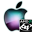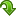ATOMS : Mathieu functions toolbox details

# Mathieu functions toolbox

Solve Mathieu equations, calculate Mathieu functions
Details
Version
4.0.61
Authors
R. Coisson, G. Vernizzi, X. K. Yang, N. O. Strelkov
Michael Baudin
Owner Organization
Parma University - DIGITEO - NRU MPEI
Maintainers
Michael Baudin
Nikolay Strelkov
Categories
Creation Date
March 28, 2020
Source created on
Scilab 6.1.x
Binaries available on
Scilab 6.1.x:Windows 64-bitWindows 32-bitLinux 64-bitLinux 32-bitMacOSX
Install command
`--> atomsInstall("Mathieu")`
Report a bugDescription
```            Purpose
-------
This Mathieu Functions Toolbox is used to solve Mathieu function numerically
.

The Mathieu equation is a second-order homogeneous linear differential equation
and appears in several different situations in Physics: electromagnetic or
elastic wave equations with elliptical boundary conditions as in waveguides or
resonators, the motion of particles in alternated-gradient focussing or
electromagnetic traps, the inverted pendulum, parametric oscillators, the motion
of a quantum particle in a periodic potential, the eigenfunctions of the quantum
pendulum, are just few examples. Their solutions, known as Mathieu functions,
were first discussed by Mathieu in 1868 in the context of the free oscillations
of an elliptic membrane.

We present both the Floquet solution and solution in angular (AMF) and radial
(modified) (RMF) functions [2-4].

The toolbox has 14 demos.
All demos can be acessed from "?->Scilab Demonstrations->Mathieu
functions"

Authors
-------
R.Coisson & G. Vernizzi, Parma University, 2001-2002.
X. K. Yang
N. O. Strelkov, NRU MPEI
Michael Baudin - DIGITEO - 2011

Features
--------
> Coordinate transformation
Almost all tasks, involving Mathieu functions have elliptical geometry. For
coordinate transformation we have functions:
* mathieu_cart2ell 	convert coordinates from Cartesian to elliptical
* mathieu_ell2cart 	convert coordinates from elliptical to Cartesian
* mathieu_cart2pol 	convert coordinates from Cartesian to polar
* mathieu_pol2cart 	convert coordinates from polar to Cartesian
* mathieu_ell_in_pol 	calculate polar coordinates of a point at known angle on
ellipse

* For elliptical coordinates we have demos "Elliptic and Cartesian
coordinates" and Tab1 of demo "GUI for Mathieu Function
Toolbox".

> Floquet solution
For Floquet solution we have the following functions:
* mathieu_mathieuf 	evaluate characteristic values and expansion coefficients
* mathieu_mathieu 	evaluate periodic Mathieu functions by calling
mathieu_mathieuf
* mathieu_mathieuexp 	calculate the characteristic exponent of non-periodic
solutions and the coefficients of the expansion of the periodic factor
* mathieu_mathieuS 	calculate solutions of ME with arbitrary a and q
parameters (uses mathieu_mathieuexp)

For Floquet solutions we have demos:
* Plots of AMFs with first derivative are available in Tab2 of demo named
"GUI for Mathieu Function Toolbox".
* Plots of ce_m(z,q), se_m(z,q) of order 0-5 for q = 1,10 for comparison with
Abramowitz and Stegun (Figs. 20.2-20.5, [3, pp. 725-726]).
* Tables of ce_m(z,q) and ce_m'(z,q) for comparison with Abramowitz and Stegun
(Table 20.1 [3, p. 748]).
* Tables of se_m(z,q) and se_m'(z,q) for comparison with Abramowitz and Stegun
(Table 20.1 [3, p. 749]).
* Plots of ce_m(z,q), se_m(z,q) of order 0-3 for q = 0-30 and z = [0, π]
(Fig. 2, [5, p. 235]).

> Solution in AMF and RMF functions:
We present solutions of Mathieu equation as AMF [2,3]:
ce_m(z,q) and se_m(z,q) 
and solutions of modified Mathieu equation (2) as RMF [2,3]:
Mc_m(1)(z,q), Ce_m(z,q), Mc_m(2)(z,q), Gey_m(z,q) and Ms_m(1)(z,q), Se_m(z,q),
Ms_m(2)(z,q), Fey_m(z,q).

Before calculating any Mathieu function we calculate expansion coefficients
and eigenvalues for given order m and parameter q using tri-diagonal matrixes
[5-8].

We have the following functions for computation solutions of Mathieu
equations:
* mathieu_Arm 	compute expansion coefficients 'Arm' and eigenvalue 'am' for
even AMF and RMF
* mathieu_Brm 	compute expansion coefficients 'Brm' and eigenvalue 'bm' for
odd AMF and RMF
* mathieu_ang_ce 	compute even AMF 'ce' or its first derivative
* mathieu_ang_se 	compute odd AMF 'se' or its first derivative
* mathieu_rad_mc 	compute even RMF 'Mc' or its first derivative (kinds 1 and
2)
* mathieu_rad_ms 	compute odd RMF 'Ms' or its first derivative (kinds 1 and
2)
* mathieu_rad_ce 	compute even RMF of the first kind 'Ce' or its first
derivative
* mathieu_rad_se 	compute odd RMF of the first kind 'Se' or its first
derivative
* mathieu_rad_fey 	compute even RMF of the second kind 'Fey' or its first
derivative
* mathieu_rad_gey 	compute odd RMF of the second kind 'Gey' or its first
derivative

During unit-testing all functions were tested against known tables: eigenvalues,
expansion coefficients and AMF and its first derivatives were compared with [3,
9], RMF and their first derivatives were compared with [9-12].

* For eigenvalues we have demo, named "Stability chart for eigenvalues of
Mathieu`s equations" (for comparison with http://dlmf.nist.gov/28.17 ).

For modified solutions we have demos:
* Plots of RMFs with first derivative are available in Tab3 of demo "GUI
for Mathieu Function Toolbox".
* Plots of Mc_m(1)(z,q), Ms_m(1)(z,q) of order 0-2 for q = 0-3 and z =[0,
π].
* Plots of Mc_m(2)(z,q), Ms_m(2)(z,q) of order 0-2 for q = 0-3 and z =[0,
π].
* Plots of Ce_m(z,q), Se_m(z,q) of order 0-2 for q = 0-3 and z = [0,π].
* Plots of Fey_m(z,q), Gey_m(z,q) of order 0-2 for q = 0-3 and z = [0,π].

All functions have examples with plots, some of them for comparison with
[3,4].
> Calculation modes of elliptical membrane

This toolbox allows elliptical membrane mode calculation. We have two functions
for this purpose:
* mathieu_rootfinder - rootfinder for RMF or its first derivative (finds q
values of given RMF type with known order m and radial argument ξ0, which
satisfies the equation RMF_m(q,xi0) = 0 (Dirichlet boundary condition) or
RMF_m'(q,xi0) = 0 (Neumann boundary condition).
* mathieu_membrane_mode - calculate elliptical membrane mode for known
semi-axes, mode numbers, mode type and boundary condition (Soft/Dirichlet or
Hard/Neumann).

During unit-testing these functions were tested against tables and plots from
[2, 13-18].

For elliptical membrane we have demos:
* Tab4 of demo "GUI for Mathieu Function Toolbox".
* Elliptic membrane: comparison of 2 even soft modes.
* Elliptic membrane: comparison of 4 even & odd, soft & hard.
* Elliptic membrane: 3D surface plot of a even soft mode with m=3, n=3.

Bibliography
------------
1. R. Coïsson, G. Vernizzi and X.K. Yang, "Mathieu functions and numerical
solutions of the Mathieu equation", IEEE Proceedings of OSSC2009 (online at
http://www.fis.unipr.it/~coisson/Mathieu.pdf).
2. N.W. McLachlan, Theory and Application of Mathieu Functions, Oxford Univ.
Press, 1947.
3. M. Abramowitz and I.A. Stegun, Handbook of Mathematical Functions, Dover, New
York, 1965.
4. Chapter 28 Mathieu Functions and Hill's Equation. Digital Library of
Mathematical Functions. NIST.
5. J. C. Gutiérrez-Vega, R. M. Rodríguez-Dagnino, M. A. Meneses-Nava, and S.
Chávez-Cerda, "Mathieu functions, a visual approach", American
Journal of Physics, 71 (233), 233-242. An introduction to applications.
6. J. J. Stamnes and B. Spjelkavik. New method for computing eigenfunctions
(Mathieu functions) for scattering by elliptical cylinders. Pure Appl. Opt. 4
251-62, 1995.
7. L. Chaos-Cador, E. Ley-Koo. Mathieu functions revisited: matrix evaluation
and generating functions. Revista Mexicana de Fisica, Vol. 48, p.67-75, 2002.
8. Julio C. Gutiérrez-Vega, "Formal analysis of the propagation of
invariant optical fields in elliptic coordinates", Ph. D. Thesis, INAOE,
México, 2000.
9. S. Zhang and J. Jin. Computation of Special Functions. New York, Wiley,
1996.
10. G. Blanch and D. S. Clemm. Tables relating to the radial Mathieu functions.
Volume 1. Functions of the First Kind. ARL, US Air Force. 1963.
11. G. Blanch and D. S. Clemm. Tables relating to the radial Mathieu functions.
Volume 2. Functions of the Second Kind. ARL, US Air Force. 1963.
12. E. T. Kirkpatrick. Tables of Values of the Modified Mathieu Functions.
Mathematics of Computation, Vol. 14, No. 70 (Apr., 1960), pp. 118-129.
13. Wilson, Howard B., and Robert W. Scharstein. "Computing elliptic
membrane high frequencies by Mathieu and Galerkin methods." Journal of
Engineering Mathematics 57.1 (2007): 41-55.
14. Neves, Armando GM. "Eigenmodes and eigenfrequencies of vibrating
elliptic membranes: a Klein oscillation theorem and numerical
calculations." Comm. Pure Appl. Anal. 2009.
15. Shibaoka, Yoshio, and Fusako Iida. "On the free oscillation of water in
a lake of elliptic boundary." The Journal of the Oceanographical Society of
Japan. 21.3 (1965): 103-108.
16. Hamidzadeh, Hamid R., and L. Moxey. "Analytical modal analysis of
thin-film flat lenses." Proceedings of the Institution of Mechanical
Engineers, Part K: Journal of Multi-body Dynamics 219.1 (2005): 55-59.
17. Lee, W. M. "Natural mode analysis of an acoustic cavity with multiple
elliptical boundaries by using the collocation multipole method." Journal
of Sound and Vibration 330.20 (2011): 4915-4929.
18. Gutiérrez-Vega, J., S. Chávez-Cerda, and Ramón Rodríguez-Dagnino.
"Free oscillations in an elliptic membrane." Revista Mexicana de
Fisica 45.6 (1999): 613-622.
```Files (2)OS-independent binary for Scilab 6.1.x
```
```Source code archive
```
```News (0)Comments (0)Leave a comment
You must register and log in before leaving a comment.Email notifications
Send me email when this toolbox has changes, new files or a new release.